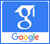# Neutrino## Fifth “dark” force completely change our understanding of the universe

Published on: 29th July, 2019

OCLC Number/Unique Identifier: 8259319214

For something that’s literally as old as the universe, dark matter doesn’t get much attention outside scientific circles. Maybe that’s because, other than a short-lived SyFy series and a late-period Randy Newman album, this nebulous star stuff has had a tough time breaking the pop-culture barrier. But the truth is that today, dark matter has never mattered more. Our own Milky Way is embedded in a massive cloud of it, we’re looking for its interactions deep inside the earth, and there are whole galaxies without it. So what is dark matter, anyway? Why can’t scientists get enough of the stuff, even though they can’t actually find it? What deep, dark secrets does it hold? And could it ultimately shape the future of life as we know it?## Oscillation of neutrino in a vacuum with mixing flavor

Published on: 30th November, -0001

We developed multiple equations to observe the two and three flavors of neutrino oscillation with the mixing angle based on L/E=0.1 to 0.9 in this study. In different settings, the nature of the neutrino oscillation probability was discovered to be varied in different equations. The observation indicates increasing likelihood in one equation and decreasing probability in the other equations in two flavor oscillation neutrinos. To characterize the probability of neutrino oscillation, we use four distinct angles: 50, 100, 150, and 200. The probability of neutrino oscillation was determined to be highest at an angle of 150 degrees. However, with increasing mixing angles, the likelihood of oscillation increases on the basis of created equation (25) and decreases on the basis of equations (26) and (27) in the three-flavor neutrino oscillation. From generated equations (25) and (26) the maximum neutrino oscillation of probability is discovered at an angle of 150, however, from equation (27), the maximum probability is observed at 50. The greatest neutrino oscillation is found to be 0.9999 and the minimum is zero in all of these two and three flavors of oscillation.## Oscillation of neutrino in a vacuum with mixing flavor

Published on: 4th August, 2022

We developed multiple equations to observe the two and three flavors of neutrino oscillation with the mixing angle based on L/E=0.1 to 0.9 in this study. In different settings, the nature of the neutrino oscillation probability was discovered to be varied in different equations. The observation indicates increasing likelihood in one equation and decreasing probability in the other equations in two flavor oscillation neutrinos. To characterize the probability of neutrino oscillation, we use four distinct angles: 50, 100, 150, and 200. The probability of neutrino oscillation was determined to be highest at an angle of 150 degrees. However, with increasing mixing angles, the likelihood of oscillation increases on the basis of created equation (25) and decreases on the basis of equations (26) and (27) in the three-flavor neutrino oscillation. From generated equations (25) and (26) the maximum neutrino oscillation of probability is discovered at an angle of 150, however, from equation (27), the maximum probability is observed at 50. The greatest neutrino oscillation is found to be 0.9999 and the minimum is zero in all of these two and three flavors of oscillation.